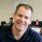# Are the Load Combinations Balanced?

In April’s post about the Omega Factor, one commenter asked of the 1.2 increase allowed by ASCE 12.4.3.3, “Why do they allow a stress increase for allowable combinations? Seems unconservative for steel now that they have essentially balanced the ASD capacity with LRFD.”

To be honest, I have never spent much time analyzing which design methodology was more or less conservative. If I was designing with wood I would use ASD, and if it was with concrete I would use LRFD. Steel was strictly ASD early on in my design career, but LRFD usage grew. The question about balance made me curious. Are the load combinations balanced?

Of course, just comparing the load combinations would be meaningless. We know the LRFD combinations result in higher design forces. But those higher forces are compared to higher design strengths. So we need to normalize things.

The AISC Specification for Structural Steel Buildings, ANSI/AISC 360-10 is a unified standard where each limit state has a nominal strength, Rn. For LRFD you multiply by a resistance factor, ɸ, to obtain the design strength, ɸRn.  Divide the nominal strength by a safety factor, Ω, to obtain the allowable strength, Rn/Ω, to use for ASD.

The values of ɸ and Ω in the AISC Specification are typically ɸ=0.9, Ω=1.67 for limit states such as flexure or shear, or ɸ=0.75, Ω=2.0 for net section rupture. In each case, however, the value of ɸΩ = 1.5, meaning the ratio of design strength to allowable strength is 1.5 for steel. This makes a comparison of the design methodologies for steel relatively simple.

The National Design Specification (NDS) for Wood Construction is somewhat similar, with Appendix N using format conversion factors, KF, to convert ASD capacities to nominal and then resistance factors to go from nominal to design strength. To account for allowable stress increases, the conversion uses ASD capacities at normal duration of load also applies a Time Effect Factor, λ, which varies by load combination.

A similar comparison can be done for wood, where ɸKF = 2.16 (ignoring Fc-perp). But factoring in load duration and time effect factors, you get a ratio of design strength to allowable strength that varies from 1.35 to 1.73 depending on your load combination.

Huh?

Maybe an example with numbers for the wood will make that more clear. For an ASD wood capacity of 1000 lbs at a Cd = 1.00. The LRFD design strength will be,

ɸKF x (ASD capacity) x (time effect factor, λ)

ASD strength = 1000 lbs (Cd = 1.00)

LRFD design strength = 2.16 x 1000 lbs x 0.8 = 1728 lbs

So the ratio of design strength to allowable strength is 1.73.

For a wind or earthquake load combination, λ = 1.0 (per NDS Table N3) but we have a load duration factor, Cd of 1.6 on allowable load. So,

ASD strength = 1.6 x 1000 lbs = 1600 lbs

LRFD design strength = 2.16 x 1000 lbs x 1.0 = 2160

The ratio of design strength to allowable strength in this case is 2160/1600 = 1.35.

To see how a comparison of the load combinations works with these ratios, I’ll pick a simple D+L load case where D=L = 1000. The load combinations would be,

(LRFD EQ 16-2)  1.2D + 1.6L = 1.2×1000 + 1.6×1000 = 2800

(ASD EQ 16-9)    D + L = 1000 + 1000 = 2000

The ratio of design strength to allowable strength is 1.5 for steel and 1.73 for wood. If we compare steel first, we have (2800/1.5) / 2000 = 0.93. For wood, we have (2800/1.73) / 2000 = 0.81. If the number is less than 1.00 then there is a bias toward LRFD and LRFD is less conservative. A number greater than 1.00 is a bias toward ASD.

The chart below is the comparison of about 350 load cases with D, L, Lr and W/E loads varying from 0 to 2000.For the cases with only gravity loads, LRFD combinations appear to give a more efficient design for most cases. Whether or not those efficiencies can be realized may depend on whether or not other limits such as deflection govern member sizes. When you add in short term wind or earthquake loading, the results are mixed and neither methodology has a clear advantage.

Do you choose between LRFD or ASD to get the most efficient design? Let us know by posting a comment.

– Paul## Author: Paul McEntee

A couple of years back we hosted a “Take your daughter or son to work day,” which was a great opportunity for our children to find out what their parents did. We had different activities for the kids to learn about careers and the importance of education in opening up career opportunities. People often ask me what I do for Simpson Strong-Tie and I sometimes laugh about how my son Ryan responded to a questionnaire he filled out that day: